# Confirmation holism

Confirmation holism, also called epistemological holism, is the claim that a single scientific theory or a single hypothesis in isolation from other background theories or hypotheses cannot be tested; a test of one theory always depends on other theories and hypotheses. A theory or a hypothesis is a part of other theories and a theory or a hypothesis can be tested only as a whole. Because the thesis was formulated by Pierre Duhem and Willard Van Orman Quine, it is also known as Duhem–Quine thesis. While Duhem limited the validity of his thesis to the realm of physics only, Quine argued that the thesis is applicable to all human knowledge including mathematics and logic.

Following the Duhem–Quine thesis, Norwood Russell Hanson developed the concept of "theory-ladenness" of observation; observation does not constitute neutral, objective data, but a theory dependent interpreted knowledge. Together with Thomas Kuhn's argument for the existence of the socio-historical dimension in scientific knowledge, the Duhem–Quine thesis made a significant contribution to the philosophy of science and theory of knowledge. Their theses also blurred the sharp distinction between theories in the natural sciences and those in the social and human sciences, distinctions that had been in question under hermeneutics.

## Duhem–Quine thesis

The Duhem–Quine thesis (also called the Duhem–Quine problem) states that it is impossible to test a scientific hypothesis in isolation, because an empirical test of the hypothesis requires one or more background assumptions (also called auxiliary assumptions or auxiliary hypotheses). The hypothesis in question is by itself incapable of making predictions. Instead, the consequences of the hypothesis typically rest on background assumptions from which to derive predictions. This prevents a theory from becoming conclusively falsified through empirical means if the background assumptions are not proven (since background assumptions sometimes involve one or more scientific theories, and scientific theories are never strictly proven).

For instance, to "disprove" the idea that the Earth was moving, some people noted that birds did not get thrown off into the sky whenever they let go of a tree branch. That datum is no longer accepted as empirical evidence that the Earth is not moving because we have adopted a different background system of physics that allows us to make different predictions.

Another example is in the first half of the nineteenth century, astronomers were observing the path of the planet Uranus to see if it conformed to the path predicted by Newton's law of gravitation. It did not. There were an indeterminate number of possible explanations, such as that the telescopic observations were wrong because of some unknown factor; or that Newton's laws were in error; or some other factors affected observational data. However, it was eventually accepted that an unknown planet was affecting the path of Uranus, and that the hypothesis that there are seven planets in our solar system was rejected. Le Verrier calculated the approximate position of the interfering planet and its existence was confirmed in 1846. We now call the planet Neptune.

Although a bundle of theories (i.e. a theory and its background assumptions) as a whole can be tested against the empirical world and be falsified if it fails the test, the Duhem–Quine thesis says it is impossible to isolate a single hypothesis in the bundle. One solution to the dilemma thus facing scientists is that when we have rational reasons to accept the background assumptions as true (e.g. scientific theories via evidence) we will have rational—albeit inconclusive—reasons for thinking that the theory tested is probably wrong if the empirical test fails.

While underdetermination does not invalidate the principle of falsifiability first presented by Karl Popper, Popper himself acknowledged that continual ad hoc modification of a theory provides a means for a theory to avoid being falsified (cf. Lakatos). In this respect, the principle of parsimony, or Occam's Razor, plays a role. This principle presupposes that between multiple theories explaining the same phenomenon, the simplest theory—in this case, the one that is least dependent on continual ad hoc modification, is to be preferred.

## Pierre Duhem

As popular as the Duhem–Quine thesis may be in philosophy of science, in reality Pierre Duhem and Willard Van Orman Quine stated very different theses. Duhem believed that only in the field of physics can a single individual hypothesis not be isolated for testing. He says in no uncertain terms that experimental theory in physics is not the same as in fields like physiology and certain branches of chemistry. Also, Duhem's conception of "theoretical group" has its limits, since he states that not all concepts are connected to each other logically. He did not include at all a priori disciplines such as logic and mathematics within the theoretical groups in physics, since they cannot be tested experimentally.

## Willard Van Orman Quine

Quine, on the other hand, in his "Two Dogmas of Empiricism," presents a much stronger version of underdetermination in science. His theoretical group embraces all of human knowledge, including mathematics and logic. He contemplated the entirety of human knowledge as being one unit of empirical significance. Hence all our knowledge, for Quine, would be epistemologically no different from ancient Greek gods, which were posited in order to account for experience. Quine even believed that logic and mathematics can also be revised in light of experience, and presented quantum logic as evidence for this. Years later he retracted this position; in his book Philosophy of Logic, he said that to revise logic would be essentially "changing the subject." In classic logic, connectives are defined according to truth values. The connectives in a multi-valued logic, however, have a different meaning than those of classic logic. As for quantum logic, it is not even a logic based on truth values, so the logical connectives lose the original meaning of classic logic. Quine also notes that deviant logics usually lack the simplicity of classic logic, and are not so fruitful.

Following Duhem and Quine, Norwood Russell Hanson (1924 – 1967) developed a concept of "theory ladenness" or "theory loadedness" of observation that observation language and theory language are deeply interwoven.

In his best-known work Patterns of Discovery (1958), Hanson argued that what we see and perceive is not what our senses receive as neutral knowledge, but is instead filtered sensory information, where the filter is our existing preconceptions—a concept later called a 'thematic framework.' He cited optical illusions such as the "My Wife and My Mother-In-Law" (Patterns of Discovery, p. 11), which can be seen in different ways. Hanson drew a distinction between 'seeing as' and 'seeing that' which became a key idea in evolving theories of perception and meaning.

## Logical formulation

### Theory-dependence of observations

Suppose some theory T implies an observation O (observation meaning here the result of the observation, rather than the process of observation per se):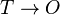$T \rightarrow O$$\sim O$

So by Modus Tollens,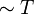$\sim T$

All observations make use of prior assumptions, which can be symbolized as: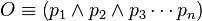$O \equiv \left( p_1 \wedge p_2 \wedge p_3 \cdots p_n \right)$

and therefore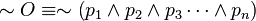$\sim O \equiv \sim \left( p_1 \wedge p_2 \wedge p_3 \cdots \wedge p_n \right)$

which is by De Morgan's law equivalent to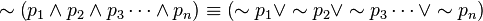$\sim \left( p_1 \wedge p_2 \wedge p_3 \cdots \wedge p_n \right) \equiv \left( \sim p_1 \vee \sim p_2 \vee \sim p_3 \cdots \vee \sim p_n \right)$.

In other words, the failure to make some observation only implies the failure of at least one of the prior assumptions that went into making the observation. It is always possible to reject an apparently falsifying observation by claiming that only one of its underlying assumptions is false; since there are an indeterminate number of such assumptions, any observation can potentially be made compatible with any theory. So it is quite valid to use a theory to reject an observation.

### Underdetermination of a theory by evidence

Similarly, a theory consists of some indeterminate conjunction of hypotheses,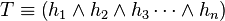$T \equiv \left( h_1 \wedge h_2 \wedge h_3 \cdots \wedge h_n \right)$

and so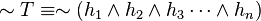$\sim T \equiv \sim \left( h_1 \wedge h_2 \wedge h_3 \cdots \wedge h_n \right)$

which implies that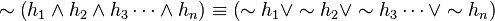$\sim \left( h_1 \wedge h_2 \wedge h_3 \cdots \wedge h_n \right) \equiv \left( \sim h_1 \vee \sim h_2 \vee \sim h_3 \cdots \vee \sim h_n \right)$

In words, the failure of some theory implies the failure of at least one of its underlying hypotheses. It is always possible to resurrect a falsified theory by claiming that only one of its underlying hypotheses is false; again, since there are an indeterminate number of such hypotheses, any theory can potentially be made compatible with any particular observation. Therefore it is in principle impossible to conclusively determine if a theory is false by reference to evidence.

## Philosophical implications

Karl Popper referred to the Duhem–Quine thesis in his The Logic of Scientific Discovery (1934), and argued that: falsification of a hypothesis is possible by avoiding ad hoc revisions, although the refutation of a theory is logically impossible as the thesis pointed out.

Thomas Kuhn cited the Duhem–Quine thesis as one of the reasons why paradigms are incommensurable.

In direct reference to the Duhem–Quine thesis, Larry Laudan argued that: indeterminary does not threaten the scientific rationality; although indeterminacy is logically possible and true, a rational choice is possible by maintaining a high degree of coherence and consistency.

These arguments raised questions regarding the nature of rationality and the status of natural science as the model of scientific rationality. If the principle of indeterminary and theory-ladenness are applicable to theories of natural sciences, the sharp distinction between natural science and social, human sciences can be questioned. In modern hermeneutics, such as Dilthey's, natural sciences were distinguished from the human, social sciences. Natural sciences are understood as non-hermeneutic, objective, purely rational disciplines, whereas the human, social sciences are hermeneutic and their rationality involves interpretation. Although those two type of disciplines have significant differences, a hermetical approach is probably possible to all forms of knowledge including the natural sciences. The question is extended to the lasting debate on religion, where lies the roots of hermeneutics, versus science.